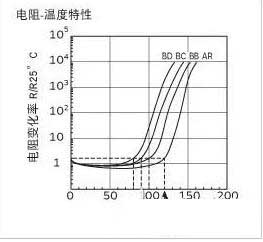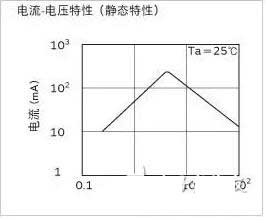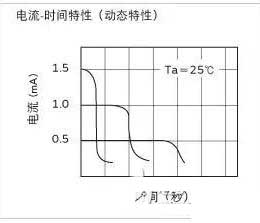﻿ Ptc thermistor temperature-characteristic curve_

China sensor manufacturers

# Ptc thermistor temperature-characteristic curve

1, resistance-temperature characteristics
Although there is a slight difference between the normal temperature and the Curie point temperature, POSISTOR® still shows almost constant resistance-temperature characteristics. Its resistance-temperature characteristic is that when the temperature exceeds the Curie point, the resistance will rise abruptly.
Curie point (C.P.) is defined as the temperature at which the resistance value is equal to twice the resistance of 25 °C.2. Current-voltage characteristics (static characteristics)
This shows the relationship between the applied voltage and the regulated current when the voltage applied to the POSISTOR® balances internal heat and external heat dissipation. It has both a current maximum point and a constant output power section.3. Current-time characteristics (dynamic characteristics)
This shows the relationship between current and time before internal heat and external heat dissipation reach equilibrium. It is characterized by a large initial current and a sudden continuous attenuation.﻿
﻿